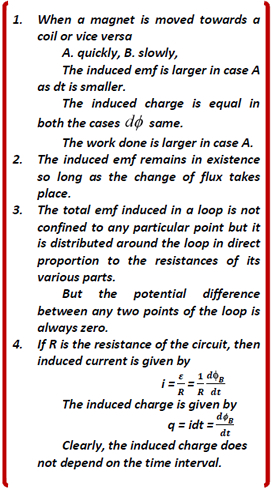Examples

View SolutionsExample 1

The magnetic flux through a loop increases according to the relation θ B = 5.0t2 + 6.0t where θB is in milliwebers and t in seconds. What is the magnitude of the emf induced in the loop when t = 2.0 s?

Example 2

A field of 5 x 10-2 Wb/m2 acts at right angles to the coil of area 0.2 m2 having 100 turns. The coil is removed from the field in 0.05 second. Find the emf induced in the coil in volt.

Example 3

A coil having an area A is placed in a magnetic field which changes from B to 3 B in time interval t. What will be the emf induced in the coil?

Example 4

A circular copper loop of radius r and resistance R is placed with its plane perpendicular to a variable magnetic field given by B = B0 sin w t. Obtain the expression for the induced current in the loop.

Example 5

The rails of a railway track separated by 1m are connected to a millivoltmeter. The rails are insulated from each other and the ground. A superfast train travels at a speed of 135 km/h. What will the reading of the millivoltmeter if the horizontal component of the Earth's magnetic field at that place is 0.2 Gauss?

Example 6

An aeroplane with a wing span of 50 m is flying horizontally with a speed is 200 m/s. The horizontal and 9 vertical components of Earth's magnetic field are 0.3 gauss and 0.5 gauss respectively. Find the potential difference between the wing tips of the aeroplane induced by its motion through the Earth's magnetic field.

Example 7

A square loop of side 10 cm and resistance 1 ohm is moved towards right with a constant velocity v as shown in figure 10. The left arm of the loop is in a uniform magnetic field of 2 T. The field is perpendicular to the plane of the figure and is coming out of it. The loop is connected to a network of resistors each of value 3 ohm. With what speed should the loop be moved so that a steady current of 1 mA flows in the loop?

Example 8

A metal rod of length l and mass m is free to slide, without friction, on two parallel metal rails. The rails are connected at one end as shown in figure 8. The rod has a resistance R, and the rails have a negligible resistance. A uniform magnetic field is perpendicular to the plane of this circuit. The magnetic field is increasing at a constant rate db/dt . Initially the magnetic field has strength B0 and the rod is at rest at a distance x0 from the connected end of the rails. Express the acceleration of the rod at this instant in terms of the given quantities.

Example 9

A cycle wheel with metal spokes of length 0.5 m is rotated in a magnetic field of 2 x 10-5 T normal to the plane of the wheel. An emf of 1 mV is induced between the rim and the axle. Find the angular velocity of the wheel.

Example 10

A rod of length 1 m aligned in east-west direction falls vertically downward at a constant speed of 72 km/h at a place where the vertical component of the Earth's magnetic field is 1/2 √3 gauss and angle of dip 300. Find the potential difference between two ends of the rod.

CBSE Electromagnetic Induction ( With Hint / Solution)
Class XII (By Mr. Ashis Kumar Satapathy)
email - [email protected]

Electromagnetic Induction